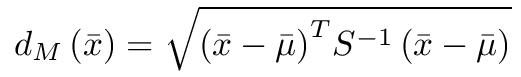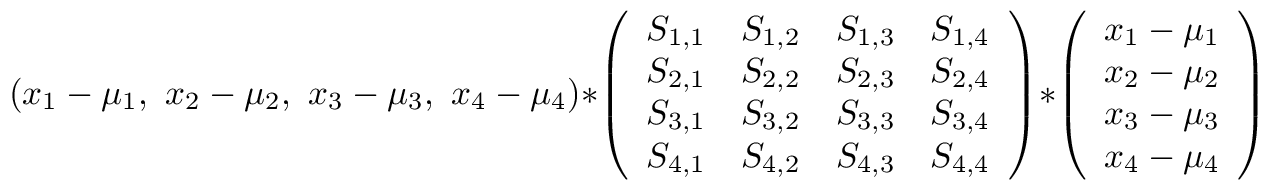## Clustering Fisher's Iris Data

The Fisher iris data, sometimes referred to as the Anderson's iris dataset, is a standard dataset provided to statistics and machine learning students. ( for a full description see the Wiki page: Iris flower data set) I first encountered this data set in 1993 during a graduate level neural network class at NC A&T SU.

Recently, I built a clustering algorithm for multi-dimensional datasets. I used the Mahalanobis distance as my similarity metric.

The Mahalanobis distance is defined as:for the purpose of a clustering metric the square root is computational overhead. If f(x) is monotonic increasing then so is sqrt[ f(x) ].There are three classes in the Iris data: Setosas, Vericolor, and Virginica. Three clusters are created. Each cluster maintains a mean vector and an inverse Covariance matrix. Each sample is compared to each of the three clusters using the Mahalanobis distance metric. The smallest distance value determines which cluster the sample belongs to.

The example below will classify 147 out of 150 samples correctly ( 98 percent )

The inverse Covariance matrices shown below are symmetric so only the lower triangle is shown. The three clusters are defined as:

### Setosa (full stats)

```   Mean = 5.006  3.428  1.462  0.246

Inv Covariance =
18.943439
-12.404826   15.570540
-4.500207    1.111079   38.776204
-4.776127   -2.104098  -17.935035  106.045906
```

### Vericolor (full stats)

```   Mean = 5.936  2.770  4.260  1.326

Inv Covariance =
9.502764
-3.676217   19.710966
-8.631712    2.116022   19.803758
6.454503  -19.480325  -26.937227   87.244794
```

### Virginica (full stats)

```   Mean = 6.588  2.974  5.552  2.026

Inv Covariance =
10.533867
-3.479726   15.875442
-9.960146    1.102689   13.405821
1.788152   -8.472851   -2.890918   19.314050
```
Full statistics for combined labeled and unlabeled